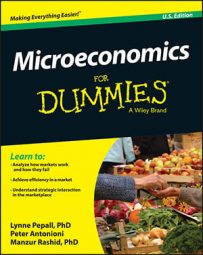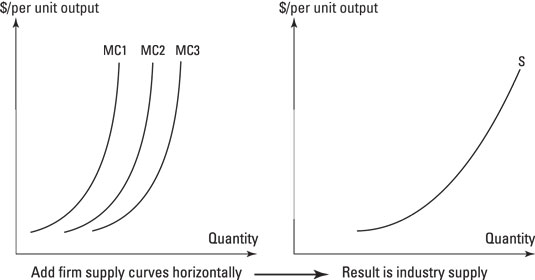##### Microeconomics For DummiesFirms in perfectly competitive markets are price takers. To understand the competitive position among the firms in a competitive market, it is helpful to look at the supply decisions an individual firm will make. This means that if you want to see what's happening in the market, you have to return to looking at the firm's cost curves.

To go from a firm's decision about how much to produce to an industry supply, you need to add up the marginal cost curves of all the firms in the industry and read off the output that's produced in aggregate at each possible price. That gets you the industry supply curve that you use to find equilibrium output.

The thing is, in perfect competition the assumption that market entry and exit for firms is costless means that supply in a perfectly competitive market looks a little different. This example of the paper clip industry shows you how horizontal addition works to get the figure for industry supply in the short run.

The table shows the output of three firms in the paper clip industry for three different values of marginal costs. At a marginal cost of 1, for instance, firm A makes 10 paper clips, B makes 11, and C makes 12. To get the industry supply in the short run, you add up the output of A, B, and C at each of the three marginal costs — so when all competitors produce at a marginal cost of 1, industry supply is 10 + 11 + 12, which equals 33.

Marginal Cost and Industry Output in the Paper Clip Industry
Firm Marginal Cost 1 2 3
Output per firm
A 10 11 13
B 11 12 15
C 12 14 17
Total 33 37 45

The supply curve for the industry gives the relationship between output and cost for the industry. Adding up the marginal costs for each of the firms provides the short-run supply curve for the industry.

You're adding horizontally so that you have to add up the output for all the firms.

Now, because firms are price takers, profit maximization means that marginal revenue is equal to price is equal to marginal cost or MR = p = MC. The following table shows industry output for three different prices. (You may notice that industry output is the same as in the bottom row of the preceding table. The difference is that you're using the marginal cost equals price relationship to make the inference that makes up the supply curve.)

Supply Curve for the Paper Clip Industry
Price 1 2 3
Industry output 33 37 45

When you plot the output for the three firms, you get the typical upward-sloping supply curve (see the following figure). Using the relationship between marginal cost and marginal revenue equal to price, you can express the profit-maximizing supply with price on the vertical axis and quantity on the horizontal axis, and lo and behold, you can say that if the market price of a box of paper clips is 3, then the industry would produce 45 boxes. In other words, the sum of the marginal costs of the firms in the industry leads to an output equal to 45 when marginal cost is equal to 3.Adding marginal costs horizontally to make industry supply.

This situation is fine for looking at most cases of industry supply and market demand, but the costless entry and exit condition in the long run adds something new to how you work out supply in the long run.

Costless market entry and exit means that in the short run, if any firm is making economic profits, then that situation will attract in the longer run a new firm to enter the market. That entry would compete away the profits made by firms in the industry. What matters in the long run, therefore, is what a firm would do when considering whether to enter the industry. Economists ponder that by considering something called the marginal firm.

Marginal, as usual in economics, means at the margin. At the margin, only one firm is deciding whether to enter the industry, stay in it, or leave it. At equilibrium, the marginal firm will have no preference between those decisions. How can that possibly be the case?

Well, given the profit-maximizing rule for firms — that production is set where marginal revenue equals marginal cost — you know that this can happen only when a firm receives exactly as much for its last unit of output as the incremental amount it cost to produce it. At equilibrium in perfect competition, therefore, economists look at the marginal revenue received by the last firm and find that it must equal the marginal cost of producing that output.

The marginal condition holds in the short run where capital (for example, production facilities) or the number of firms is fixed and in the long run when the condition refers to the last firm to enter the industry.